## Linear Equations

Linear sentences in one variable may be equations or inequalities. What they have in common is that the variable has an exponent of 1, which is understood and so never written (except for teaching purposes). They also can be represented on a graph in the form of a straight line.

An equation is a statement that says two mathematical expressions are equal. A linear equation in one variable is an equation with the exponent 1 on the variable. These are also known as first‐degree equations, because the highest exponent on the variable is 1. All linear equations eventually can be written in the form ax + b = c, where a, b, and c are real numbers and a ≠ 0. It is assumed that you are familiar with the addition and multiplication properties of equations.

• Addition property of equations: If a, b, and c are real numbers and a = b, then a + c = b + c.

• Multiplication property of equations: If a, b, and c are real numbers and a = b, then ac = bc.

The goal in solving linear equations is to isolate the variable on either side of the equation by using the addition property of equations and then to use the multiplication property of equations to change the coefficient of the variable to 1.

##### Example 1

Solve for x: 6(2 x – 5) = 4(8 x + 7).To isolate the x's on either side of the equation, you can either add –12 x to both sides or add –32 x to both sides.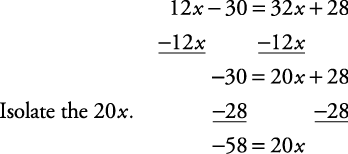Multiply each side by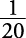(or divide each side by 20).The solution is. This is indicated by placing the solution inside braces to form a set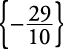. This set is called the solution set of the equation. You can check this solution by replacing x with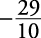in the original equation. The solution set is.

##### Example 2

Solve for x: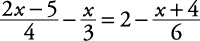.

This equation will be made simpler to solve by first clearing fraction values. To do this, find the least common denominator (LCD) for all the denominators in the equation and multiply both sides of the equation by this value, using the distributive property.Don't forget that the –2 is distributed over both the x and the 4. Simplify both sides by combining like terms.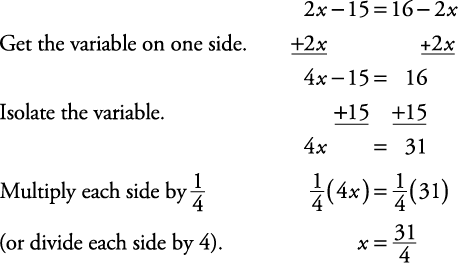You can check this for yourself. The solution set is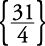.

Back to Top
A18ACD436D5A3997E3DA2573E3FD792A